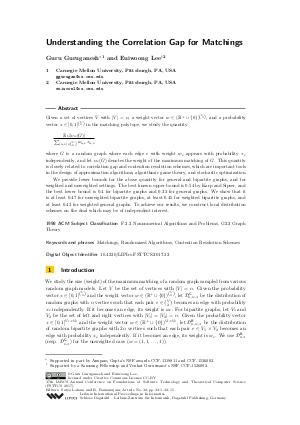Document# Understanding the Correlation Gap For Matchings

### Authors Guru Guruganesh, Euiwoong Lee## File

LIPIcs.FSTTCS.2017.32.pdf
• Filesize: 0.5 MB
• 15 pages

## Cite As

Guru Guruganesh and Euiwoong Lee. Understanding the Correlation Gap For Matchings. In 37th IARCS Annual Conference on Foundations of Software Technology and Theoretical Computer Science (FSTTCS 2017). Leibniz International Proceedings in Informatics (LIPIcs), Volume 93, pp. 32:1-32:15, Schloss Dagstuhl - Leibniz-Zentrum für Informatik (2018)
https://doi.org/10.4230/LIPIcs.FSTTCS.2017.32

## Abstract

Given a set of vertices V with |V| = n, a weight vector w in (R^+ \cup {0})^{\binom{V}{2}}, and a probability vector x In [0, 1]^{\binom{V}{2}} in the matching polytope, we study the quantity (\E_{G}[ \nu_w(G)])/(sum_(u, v) in \binom{V}{2} w_{u, v} x_{u, v}) where G is a random graph where each edge e with weight w_e appears with probability x_e independently, and let \nu_w(G) denotes the weight of the maximum matching of G. This quantity is closely related to correlation gap and contention resolution schemes, which are important tools in the design of approximation algorithms, algorithmic game theory, and stochastic optimization. We provide lower bounds for the above quantity for general and bipartite graphs, and for weighted and unweighted settings. The best known upper bound is 0.54 by Karp and Sipser, and the best lower bound is 0.4. We show that it is at least 0.47 for unweighted bipartite graphs, at least 0.45 for weighted bipartite graphs, and at least 0.43 for weighted general graphs. To achieve our results, we construct local distribution schemes on the dual which may be of independent interest.
##### Keywords
• Mathings
• Randomized Algorithms
• Correlation Gap

## Metrics

• Access Statistics
• Total Accesses (updated on a weekly basis)
0

## References

1. Shipra Agrawal, Yichuan Ding, Amin Saberi, and Yinyu Ye. Price of correlations in stochastic optimization. Operations Research, 60(1):150-162, 2012.2. Jonathan Aronson, Alan Frieze, and Boris G Pittel. Maximum matchings in sparse random graphs: Karp-sipser revisited. Random Structures and Algorithms, 12(2):111-177, 1998.3. Tom Bohman and Alan Frieze. Karp-sipser on random graphs with a fixed degree sequence. Combinatorics, Probability and Computing, 20(05):721-741, 2011.4. Prasad Chebolu, Alan M. Frieze, and Páll Melsted. Finding a maximum matching in a sparse random graph in O(n) expected time. J. ACM, 57(4):24:1-24:27, 2010. URL: http://dx.doi.org/10.1145/1734213.1734218.
5. Chandra Chekuri, Jan Vondrák, and Rico Zenklusen. Submodular function maximization via the multilinear relaxation and contention resolution schemes. SIAM J. Comput., 43(6):1831-1879, 2014. URL: http://dx.doi.org/10.1137/110839655.
6. Marek Cygan, Fabrizio Grandoni, and Monaldo Mastrolilli. How to sell hyperedges: the hypermatching assignment problem. In Proceedings of the Twenty-Fourth Annual ACM-SIAM Symposium on Discrete Algorithms, pages 342-351. Society for Industrial and Applied Mathematics, 2013.7. P Erdős and A Rényi. On random matrices ii. Studia Sci. Math. Hungar., 3:459-464, 1968.8. P Erdős and Alfréd Rényi. On the existence of a factor of degree one of a connected random graph. Acta Mathematica Hungarica, 17(3-4):359-368, 1966.9. Alan Frieze. Perfect matchings in random bipartite graphs with minimal degree at least 2. Random Structures and Algorithms, 26(3):319-358, 2005.10. Alan Frieze and Boris Pittel. Perfect matchings in random graphs with prescribed minimal degree. In Mathematics and Computer Science III, pages 95-132. Springer, 2004.11. Richard M Karp and Michael Sipser. Maximum matching in sparse random graphs. In Foundations of Computer Science, 1981. SFCS'81. 22nd Annual Symposium on, pages 364-375. IEEE, 1981.X

Feedback for Dagstuhl Publishing